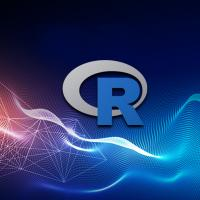Using R for Data Science: Machine Learning | TechWell

# Using R for Data Science: Machine LearningR is a system for statistical computing and graphics and is commonly used in data science. R is one of the fastest growing languages based on recent TIOBE rankings. R consists of a language, a runtime environment, and a debugger. R programs stored as scripts may be run on the command line or in an IDE such as RStudio. Machine learning in data science involves data preparation and data modeling, each of which could be further categorized into sub-tasks. Data preparation involves data extraction, transformation, and loading (ETL). For modeling, a dataset is typically split into a training dataset and a test dataset.

The training dataset comprises the majority of the original dataset, and the test dataset is only about 10–20 percent of the dataset. The training dataset is used for training a data model, and the test dataset is used for testing the model. Training a model involves selecting an algorithm, selecting features, and developing a model itself. Features are variables or attributes in a dataset that are the most likely predictors in the model being developed. Feature selection is needed as a dataset and may include features that are irrelevant or redundant as predictors of a model.

Various types of algorithms are used in data modeling, and these are grouped as supervised learning and unsupervised learning algorithms. Supervised learning involves using a labeled training dataset with a target predictor. As an example, a training dataset could consist of paired (x,y) values in which the value y is predicted based on the value of x. Such a dataset is called a labeled training dataset with the target predictor being y. Examples of Supervised learning models are Regression and Classification. Unsupervised learning does not provide any such labels. Unsupervised learning involves finding patterns in a dataset without the use of any predefined variables as predictors. An example of unsupervised learning is Clustering. After a model is developed using the training dataset, it is validated using the test dataset.
R provides some builtin packages including base, compiler, datasets, and graphics. The pre-built packages only provide the base functionality and would not suffice when R is used for data science. Add-on packages are required when using R for data science. Here are some of the packages that can be used for data science.

Data Preparation
The dataPreparation tool could be used for automated data preparation. The plyr package is used for splitting, applying, and combining data. The splitTools package provides a variety of tools for splitting data. Data could be split into groups for training, validation, and test.

Feature Selection
FSinR is a package for feature subset selection. The vscc package is used for variable selection for clustering and classification. The splitSelect package provides functions to group features or variables and samples from the feature groups. As feature selection is based on the model type, different packages are available for different types of models. The MDFS package is used for multi-dimensional feature selection. The EFS package is used for Ensemble Feature selection in which an ensemble of feature selection methods are used to find the importance of a feature with respect to a classification variable.

Regression
The rms model in R could be used for testing regression modeling strategies. Regression modeling involves predicting a continuous value from input features. The blorr package is used to build and validate binary logistic regression models. The apricom package provides tools for the comparison of regression modeling strategies. FWDSelect is a tool for selecting variables in a regression model. The gnlm is a package that provides functions to develop linear and non-linear regression models. The cvTools is a package with cross-validation tools for regression models.

Classification
Classification models in machine learning are used to predict into which class or category a new data point should be classified. The RTextTools is a package for automatic text classification via Supervised learning, and it is suitable as a starter package if you are not too familiar with classification. The regtools package provides tools for regression and classification. The caretpackage is used for training classification and regression models. The class function provides various functions for classification. The alookr package is used for predictive modeling to develop a Binary Classification model; a binary classification model has only two classes or categories. It includes tools for data splitting, predictive modeling, and model evaluation.

Clustering
Clustering is a non-supervised machine learning technique and involves grouping data points based on similarity. No labels are used in clustering. The k-means is one of the most commonly used algorithms in clustering, and R provides several packages for k-means such as ClusterR and kselection. The skm package is used for selective k-means, and the wskm is used for weighted k-means. The supcluster package is for Supervised cluster analysis. Some other clustering packages are ClustBlock,Clustering, and clusterSim.

Machine learning involves developing mathematical models using sample data and is further classified into supervised learning and unsupervised learning. Supervised learning uses labeled data to predict the target label for an input data with common examples being regression and classification. Unsupervised learning finds patterns in datasets based on similarity in data with an example being clustering.

July 29, 2020

## TechWell Insights To Go

(* Required fields)